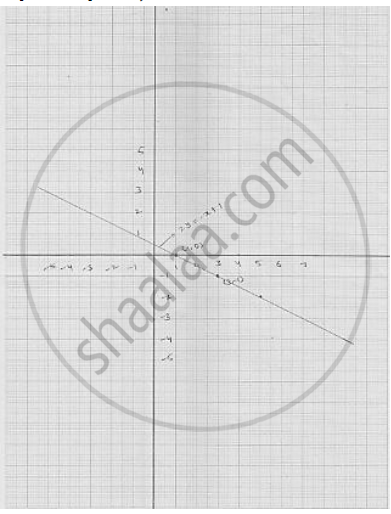# Draw the graph of the following linear equations in two variable : 2𝑦 = −𝑥 + 1 - Mathematics

Draw the graph of the following linear equations in two variable : 2𝑦 = −𝑥 + 1

#### Solution

we have

2y = -x + 1

⇒ x -1 = 2y          ............. (1)

Putting  y = 0 , we get   x = 1 - 2 × 0 = 1

Putting  y = -1 , we get  x = 1 - 2 ( - 1) = 3

Thus, we have the following table giving the two points on the line represented by
the equation

2y = x - 3

2y = - x + 1

Graph for the equation 2y = - x +1Concept: Graph of a Linear Equation in Two Variables
Is there an error in this question or solution?

#### APPEARS IN

RD Sharma Mathematics for Class 9
Chapter 7 Linear Equations in Two Variables
Exercise 7.3 | Q 1.8

Share